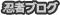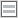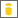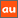# 0除算に関するアナウンスメントを公開しています

The purpose of this site is to publish announcements on the theory of division by zero

## Announcement 409: Various Publication Projects on the Division by Zero

Institute of Reproducing Kernels
Kawauchi-cho, 5-1648-16,
Kiryu 376-0041, Japan
January 29, 2018

The Institute of Reproducing Kernels is dealing with the theory of division
by zero calculus and declares that the division by zero was discovered as 0/0 =
1/0 = z/0 = 0 in a natural sense on 2014.2.2. The result shows a new basic
idea on the universe and space since Aristoteles $($BC384 - BC322$)$ and Euclid
$($BC 3 Century - $)$, and the division by zero is since Brahmagupta $($598 - 668
?$)$. In particular, Brahmagupta defined as 0/0 = 0 in Brhmasphuasiddhnta
$($628$)$, however, our world history stated that his definition 0/0 = 0 is wrong
over 1300 years, but, we showed that his definition is suitable. For the details,
see the references and the site: http://okmr.yamatoblog.net/
We wrote two global book manuscripts  with 154 pages and  with
many figures for some general people. Their main points are:

• The division by zero and division by zero calculus are new elementary
and fundamental mathematics in the undergraduate level.
• They introduce a new space since Aristoteles $($BC384 - BC322$)$ and
Euclid $($BC 3 Century - $)$ with many exciting new phenomena and
properties with general interest, not specialized and difficult topics.
However, their properties are mysterious and very attractive.
• The contents are very elementary, however very exciting with general
interest.
• The contents give great impacts to our basic ideas on the universe and
human beings.

Meanwhile, the representations of the contents are very important and
delicate with delicate feelings to the division by zero with a long and myste-
rious history. Therefore, we hope the representations of the division by zero
as follows:

• Various book publications by many native languages and with the au-
thor’s idea and feelings.
• Some publications are like arts and some comic style books with pic-
tures.
• Some T shirts design, some pictures, monument design may be consid-
ered.

The authors above may be expected to contribute to our culture, educa-
tion, common communications and enjoyments.
For the people having the interest on the above projects, we will send our
book sources with many figure files.
How will be our project introducing our new world since Euclid?
Of course, as mathematicians we have to publish new books on
Calculus, Differential Equations and Complex Analysis, at least and soon,
in order to correct them in some complete and beautiful ways.
Our topics will be interested in over 1000 millions people over the world
on the world history.

References
 M. Kuroda, H. Michiwaki, S. Saitoh, and M. Yamane, New mean-
ings of the division by zero and interpretations on 100/0 = 0 and
on 0/0 = 0, Int. J. Appl. Math. 27(2) $($2014$)$ 191-198, DOI:
10.12732/ijam.v27i2.9.
 T. Matsuura and S. Saitoh, Matrices and division by zero z/0 = 0,
Advances in Linear Algebra & Matrix Theory, 6$($2016$)$, 51-58 Published
Online June 2016 in SciRes. http://www.scirp.org/journal/alamt
http://dx.doi.org/10.4236/alamt.2016.62007.
 T. Matsuura and S. Saitoh, Division by zero calculus and singular inte-
grals $($Submitted for publication$)$.
 T. Matsuura, H. Michiwaki and S. Saitoh, $\log0=\log\infty=0$ and
applications. Differential and Difference Equations with Applications.
Springer Proceedings in Mathematics & Statistics.
 H. Michiwaki, S. Saitoh and M.Yamada, Reality of the division by zero
z/0 = 0. IJAPM International J. of Applied Physics and Math. 6 $($2015$)$,
1–8. http://www.ijapm.org/show-63-504-1.html
 H. Michiwaki, H. Okumura and S. Saitoh, Division by Zero z/0 = 0 in
Euclidean Spaces, International Journal of Mathematics and Computa
tion, 28$($2$)$$($2017$)$ 1-16.
 H. Okumura, S. Saitoh and T. Matsuura, Relations of 0 and ∞, Journal
of Technology and Social Science $($JTSS$)$, 1$($2017$)$ 70-77.
 H. Okumura and S. Saitoh, The Descartes circles theorem and division
by zero calculus. https://arxiv.org/abs/1711.04961 $($2017.11.14$)$.
 H. Okumura, Wasan geometry with the division by 0.
https://arxiv.org/abs/1711.06947 International Journal of Geometry.
 H. Okumura and S. Saitoh, Applications of the division by zero calculus
to Wasan geometry. $($Submitted for publication$)$.
 S. Pinelas and S. Saitoh, Division by zero calculus and differential equa-
tions. Differential and Difference Equations with Applications. Springer
Proceedings in Mathematics & Statistics.
 H. G. Romig, Discussions: Early History of Division by Zero, American
Mathematical Monthly, Vol. 31, No. 8. $($Oct., 1924$)$, pp. 387-389.

PR

### コメント

お名前 Black LimeGreen SeaGreen Teal FireBrick Tomato IndianRed BurlyWood SlateGray DarkSlateBlue LightPink DeepPink DarkOrange Gold DimGray Silverプロフィール

ＨＮ：